OptiSystem can optimize parameters in order to maximize, minimize or to target a value for results. This can be done by either using MPO or SPO optimizations.

By using the optimization tool you can, for example,

• optimize the fiber length of the EDFA to obtain the maximum gain
• calculate the attenuation/gain to obtain a target Q-Factor
• minimize the BER by optimizing the fiber length of the link.

In this example we show optimization of a single parameter by using SPO. It shows how to estimate the system margin. This margin shows the amount of power penalty that may be added to the system to get a defined Q factor or BER. Our target BER is 10-9, or a Q-Factor of 6.The project is given in System margin.osd, shown in Figure 1.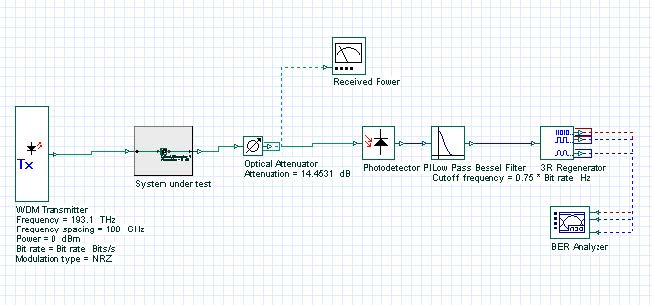Figure 1: Project layout

In this project, for illustrative purposes, the subsystem System under test is an empty system. The optimization will optimize the attenuation parameter of the Attenuator component to attain Max. Q-Factor of 6. The parameter attenuation will be the system margin in dB.

To set up the optimization, go to Tools|Optimizations… and insert a SPO Optimization. Then, select Gain Attaining type of optimization in the Main tab and set Result tolerance to 0.05. In the Parameter tab, add Attenuation of the Attenuator component into the Selected list. In the Result tab, add Max. Q factor of the BER Analyzer into the Selected list.

Figure 2, Figure 3, and Figure 4 show the Main, Parameter and Result tabs of the optimization set-up.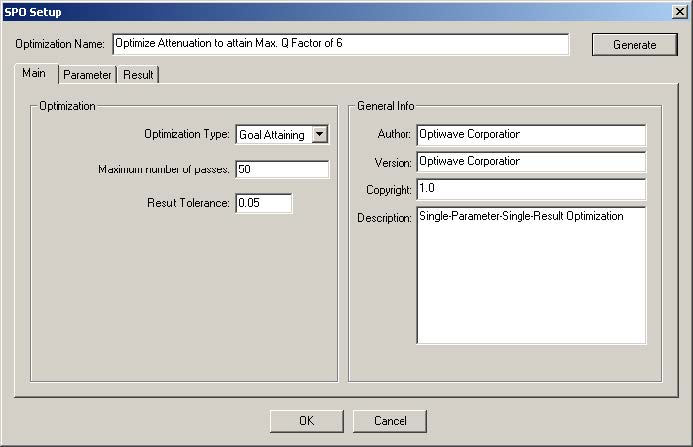Figure 2: Main tab of the SPO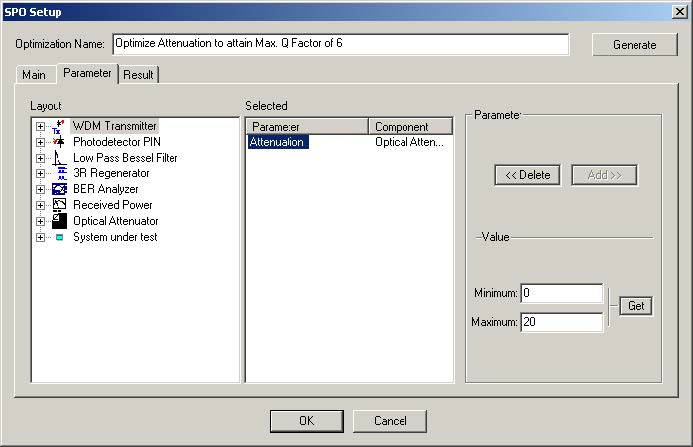Figure 3: Parameter tab of the SPO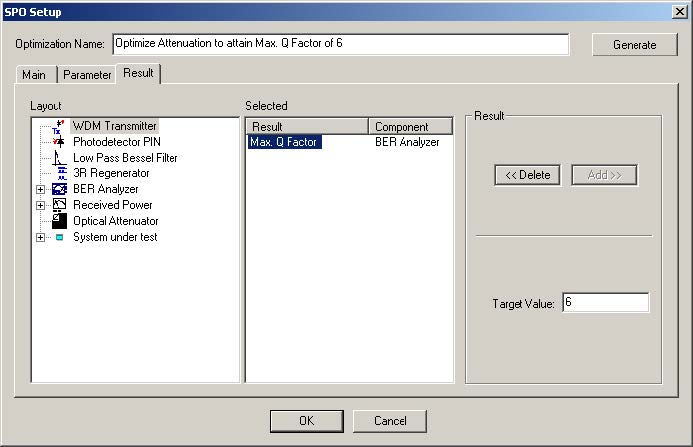Figure 4: Result tab of the SPO

In order to run the optimizations, go to File > Calculate in the Main menu, and select the Run optimizations in the Calculation dialog box, which also runs the simulation.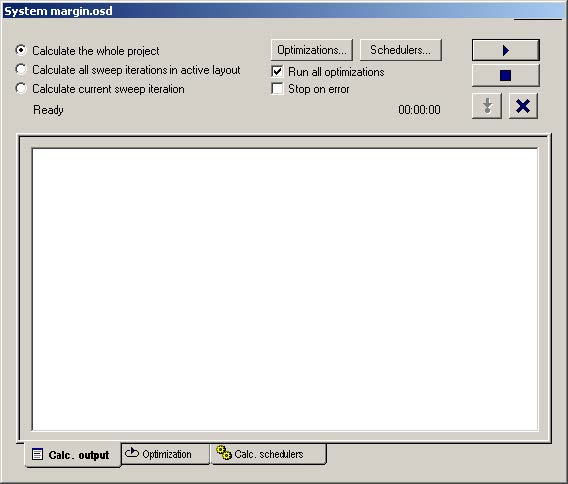Figure 5: Enabling optimizations

After the optimization is done, you will see that the system margin is approximately 19 dB for a Q-Factor of 6 (see Figure 6).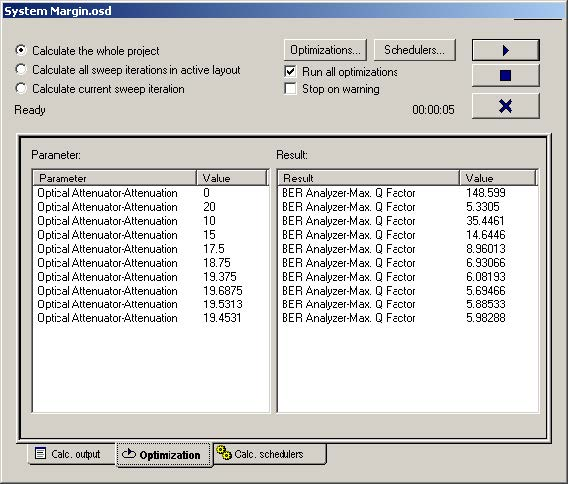Figure 6: Optimization results